• latex伪代码模板
万次阅读
2016-02-19 15:25:55

\usepackage[ruled,vlined,linesnumbered]{algorithm2e}

还可以使用；

\begin{algorithm}
\caption{Plane Distance Calculation}
\KwIn{$r_i$ , $Backgrd(T_i)$=${T_1,T_2,\ldots,T_n}$ and similarity threshold $\theta_r$}
\KwOut{$con(r_i)$}
$con(r_i)= \Phi$\;
\For{$j=1;j \le n;j \ne i$}
{
float $maxSim=0$\;
$r^{maxSim}=null$\;
\While{not end of $T_j$}
{
compute Jaro($r_i,r_m$)($r_m \in T_j$)\;
\If{$(Jaro(r_i,r_m) \ge \theta _r) \wedge ((Jaro(r_i,r_m) \ge r^{maxSim})$}
{
replace $r^maxSim$ with $r_m$\;
}
}
$con(r_i)=con(r_i) \cup {r^{maxSim}}$\;
}
return $con(r_i)$\;
\end{algorithm}

\usepackage[linesnumbered,boxed]{algorithm2e}

这个是把代码用box框住。

一、如何修改算法的标题为中文“算法”？
\renewcommand{\algorithmcfname}{算法} 即可
二、如何去掉算法中的竖线？
\SetAlgoNoLine 放在算法中即可。

参考：

http://blog.sina.com.cn/s/blog_5e16f1770100lp7u.html

更多相关内容
• Algorithm2e is an environment for writing algorithms in L ATEX2e. An algorithm is defined as a floating object like figures. It provides macros that allow you to create different sorts of key words, ...
• % ============================================= % % 确定文档类型 \documentclass{article}% % ruled 是让标题显示在上面，否则算法的标题则在下面 % linesnumbered 让算法中显示行号 % vlined 有无end ...

https://www.ctan.org/pkg/algorithm2e

% ============================================= %
% 确定文档类型
\documentclass{article}%
% ruled 是让标题显示在上面，否则算法的标题则在下面
% linesnumbered 让算法中显示行号
% vlined 有无end
% noline 没有对齐线
% 参考：https://blog.csdn.net/niekai01/article/details/52755971
\usepackage[ruled,linesnumbered]{algorithm2e}
% 设置页边距
% 参考：https://blog.csdn.net/robert_chen1988/article/details/52746354
\usepackage{geometry}
\geometry{a4paper,scale=0.8}
% 设置1.5倍行距
% ============================================= %
\begin{document}
% 是否加竖线
% \SetAlgoNoLine
\begin{algorithm}[H]
% 标题
\caption{Simulation-optimization heuristic}
% \KwData{}<====>\KwResult{}
% \KwIn{}<=======>\KwOut{}
% Data
\KwData{current period $t$, initial inventory $I_{t-1}$, initial capital $B_{t-1}$, demand samples}
% Result
\KwResult{Optimal order quantity $Q^{\ast}_{t}$}
% 空一行
% \BlankLine
% ============================================= %
% 基础语法：https://zhuanlan.zhihu.com/p/166418214
initialization\;
$r\leftarrow t$\;
$\Delta B^{\ast}\leftarrow -\infty$\;
\While{$\Delta B\leq \Delta B^{\ast}$ and $r\leq T$}
{$Q\leftarrow\arg\max_{Q\geq 0}\Delta B^{Q}_{t,r}(I_{t-1},B_{t-1})$\;
$\Delta B\leftarrow \Delta B^{Q}_{t,r}(I_{t-1},B_{t-1})/(r-t+1)$\;
\If{$\Delta B\geq \Delta B^{\ast}$}
{$Q^{\ast}\leftarrow Q$\;
$\Delta B^{\ast}\leftarrow \Delta B$\;}
$r\leftarrow r+1$\;}
\For{tiaojian}{xunhuanyuju}
\end{algorithm}
\end{document}
% 注释：\tcc是/* */注释, \tcp是//注释. 在if句使用注释时, \If(注释){条件}
% 数学公式：
% 公式居中，需要包\usepackage{amsmath}，嵌套 \begin{aligned} …& &… \end{aligned}

展开全文linux windows 运维
• 首先说一下latex关于排版的内部原理，然后说一下关于latex算法排版包的介绍，接着说一下常用的两种排版代码，最后讲一下排版的一些注意事项

## 首先说一下latex关于排版的内部原理

latex的文档由两样元素组成

1. 字符文本，就是我们写进去的文字内容
2. 浮动体，默认 figure 和 table，但是我们可以定义额外的浮动体类型，比如algorithm 宏包定义了用于排版算法的浮动体。用户也可以在导言区定义自己需要的浮动体类型（借助 float 宏包）。
（1）浮动体是一个容器（不会被分开），用于装入我们不想被分页的内容
（2）有一个简短的描述，比如标题 \caption{xx}
（3）有一个编号，用于引用，比如 \label{xx}

latex从xx.tex文件的前往后逐个字符读取内容，格式控制符解析，进行相应的输出显示。为了效率，采用的贪心算法，是不回溯的。纯字符文本内容，没什么好说的，但是遇到浮动体的时候，怎么办呢，文档一般讲究排版美学，所以浮动体会尽量显示到特定的浮动区域：

1. 在同一栏（column）当中，LaTeX 设置了两个浮动区域：栏的顶部和底部。对于双栏排版来说，LaTeX 还提供了额外的区域：跨过双栏的顶部。
2. 也有所谓的「浮动栏」或者「浮动页」的设定。顾名思义，浮动栏和浮动页就是「只有浮动体」的栏（一整栏都放多个浮动体）或者页（一整页都放多个浮动体），那么这些栏或者页，就不会放其它字符文本内容了。
3. 也可以将浮动体放在文本内容的中间（当然，这需要显式指定）。就是前后都可以有字符文本。

latex会为浮动体指定默认浮动区域（位置选项）

• ! 表示忽略一些严格的限制条件；比如忽略当前浮动区域允许放置浮动体的最大数量、当前浮动区域的最大面积，因此h选项更有可能生效（即 [!h]），所以排版出来就会比较丑，所以我们要慎用这个选项
• h 表示如有可能（比如所剩的页面还够用于当前浮动体），则放在当前位置here；
• t 表示该浮动体允许置于栏的顶部；
• b 表示该浮动体允许置于栏的底部；
• p 表示该浮动体允许置于浮动栏或浮动页。

我们有时候为了自己控制浮动体的位置，可以显式的传入位置选项（那么latex就不采用默认了），可以组合的传入（组合顺序没有影响，因为latex内部判断程序已经指定好了执行顺序，!,h,t,b,p）。

浮动算法的基本流程，可以大致描述如下。

• 当 LaTeX 遇到一个浮动体，它会根据浮动算法的规则（我们显式指定的 !,h,t,b,p 位置选项）尽可能快地输出该浮动体。
• 若成功，则该浮动体被输出，并且 LaTeX 再也不会改变它的位置。
• 若失败，则该浮动体被 LaTeX 放在一个等待队列中暂存，而后在下一页开始的时候尝试输出队列中的浮动体。
• 当一栏或者一页组装完毕，LaTeX 会检查等待队列中的浮动体，并尝试构建一个浮动栏或浮动页，输出尽可能多的浮动体。如果等待队列中的各个类型的浮动体，都不允许输出在浮动栏或浮动页中，则 LaTeX 会开始尝试将剩下的浮动体放在栏的顶部或者底部。如果当前页已无法容纳更多浮动体，则余下的浮动体又被加入等待队列，待输出下一页时再做尝试。
• 之后，LaTeX 开始处理当前页的文本信息。当然，在这个过程中，LaTeX 可能会遇到新的浮动体。
• 当 LaTeX 遇到 \clearpage/FloatBarrier 或者文档末尾时，LaTeX 会新建一个页面，而后将等待列表中的所有浮动体，都输出在浮动栏或浮动页中（而不论这些浮动体的位置选项是否指定了 p）。

注意：h 真的只表示「如果可能的话，放在这里」，如前所述，h 选项仅在一种情况下可能生效：等待列表中没有该类型的浮动体，并且当前页有足够的空间供其摆放

如果用户希望表达「我一定要放在这里」，那么需要使用 float 宏包（\usepackage{float}）提供的 H 选项。此时彻底禁止某个浮动体的浮动效果，其它位置选项h,t,p等都会无效了，如果也指定了，直接运行报错。

参考文章：

LaTeX 中的浮动体：浮动算法 | 始终

Latex：图片排版的位置参数【htbp】_weixin_39450145的博客-CSDN博客_htbp

## 然后说一下关于latex算法排版包的介绍

一般会接触到的包有algorithm、algorithmic、algorithmicx、algpseudocode、algorithm2e这5个包。

algorithm用于给伪代码提供一个浮动体容器，从而不会被分页显示。

algorithmic用于编辑伪代码的内容，一些for、while、if等语句通过该包中的命令进行编写。

algorithmicx可以看作algorithmic的升级版，提供了一些自定义命令

algpseudocode是algorithmicx的布局layout辅助
algorithm2e则是独立于algorithmic和algorithmicx的另一套伪代码环境，两套环境语法、排版上均不相同

下面是需要导入的包的3种情况：

• algorithm + algorithmic：第一代算法排版包，用的人多
• algorithm + algorithmicx + algpseudocode（内部已经导入了algorithmicx包）：第二代算法排版包，用的人也多。显示效果和第一代一样的。但是支持注释，建议用这个
• algorithm2e：第三代算法排版包，用的人少也支持注释

参考文章：

LaTeX伪代码写法总结_Zerg_Wang的博客-CSDN博客_latex 伪代码

Latex 算法怎么写？（一）：algorithm, algorithmic算法包到底什么区别？ - 知乎

上面虽然有三种使用情况，我们发现实际上第二种和第一种显示效果一样的，因此论文中最常见到的就是第二种和第三种，这两种风格：（下面的代码都是overleaf（一个在线latex编写网站，很出名）能运行通过的）

## 1. 不能带竖线的形式（用的人多）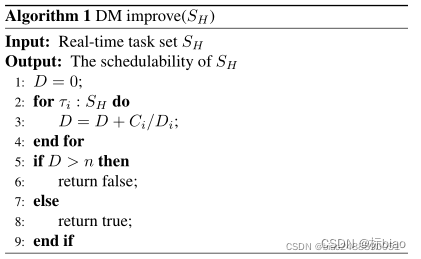latex代码如下：

%这种形式需要在前面 导入包（第二代）
\usepackage{algorithm}
\usepackage{algpseudocode}
%或者替换为\usepackage{algorithmic}，就是第一代了，
%但是关键字全部是大写形式，比如 \State 改为 \STATE

%将官方的 require 和 ensure 关键字换成我们常用的 input 和 output
\renewcommand{\algorithmicrequire}{\textbf{Input:}}
\renewcommand{\algorithmicensure}{\textbf{Output:}}

%排版代码为
\begin{algorithm}[h]
\caption{bool DM improve($S_H$)}
\begin{algorithmic}
\Require
Real-time task set $S_H$
\Ensure
The schedulability of $S_H$

\State $D=0$;

\For {${\tau _i}:{S_H}$}          %For循环结构
\State $D = D+C_i/D_i$;
\EndFor
\If {$D > n$}
\State return  false;
\Else
\State return true;
\EndIf

\end{algorithmic}
\end{algorithm}

想要添加注释，需要宏包
\usepackage{algpseudocode}
也就是，第二代排版，才支持注释

然后排版代码添加 \Comment{step 1}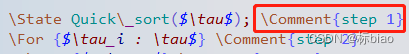效果如下：会自动右对齐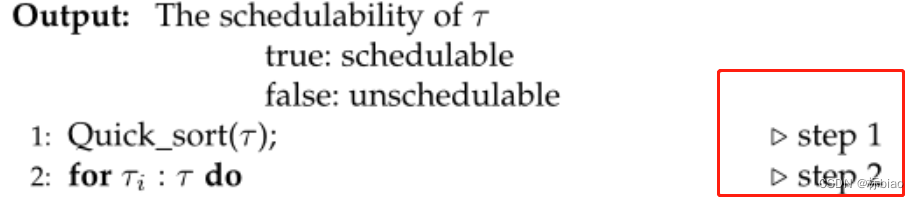## 2. 可以带竖线的形式（我觉得更好看）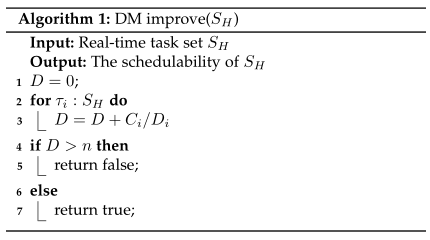latex代码如下：

%防止与已有包冲突
\makeatletter
\newif\if@restonecol
\makeatother
\let\algorithm\relax
\let\endalgorithm\relax

%引入伪代码模块需要的包，第三代
\usepackage[linesnumbered,ruled,vlined]{algorithm2e}%[ruled,vlined]{

%\usepackage[ruled]{algorithm2e} %带竖线
%\usepackage[ruled,vlined]{algorithm2e} %带竖线和折线
%\usepackage[linesnumbered,boxed]{algorithm2e} %方框格式
%\usepackage[lined,algonl,boxed]{algorithm2e} %可以显示EndIf等

%排版代码如下
\begin{algorithm}[H] %如果不能显示，这里要把[H]给加上就行了“H”是指定伪代码浮动体的位置
\caption{bool DM improve($S_H$)}
\KwIn{Real-time task set $S_H$}
\KwOut{The schedulability of $S_H$}

$D=0$\;
\For{${\tau _i}:{S_H}$}
{
$D = D+C_i/D_i$
}
\If{$D > n$}
{
return  false\;
}
\Else
{
return  true\;
}
\end{algorithm}

添加注释，只需要用下面的命令即可

\tcp{not aligned comment}，不需要引入其它包或者命令

\If(\tcp*[h]{not aligned comment}){${R_i}$},，有If时候加这儿如果想和上面第一种一样，都是自动右对齐或者左对齐，都需要额外引入一些命令，我感觉挺麻烦的，有兴趣的自己去试试吧。（因为我们手动敲空字符去实现对齐，也是可以实现的，就不整那些复杂的了。）

自动右对齐：

latex - Algorithm left margin and Comment Customizing - Stack Overflow

注意点：

• 这两种形式不能共存，会报错冲突的，因此导入的包只能选一种方式，即 \usepackage[ruled]{algorithm2e} 和包 \usepackage{algorithm}，\usepackage{algorithmic}或者\usepackage{algpseudocode} 会冲突，选其一
• 有些期刊的模板，只有一种能运行通过，且成功显示。不然要么编译始终报错，要么就是运行后算法显示不出来。这两种情况我都遇到过，深有体会。这时候记得换另外一种算法排版，一般都能解决了。
• 使用第一种伪代码模板，我最开始遇到了 ! Undefined control sequence.报错，这是因为latex代码中，该伪代码模板中关键字要么全部使用大写，要么全部大写开头而已，即，一定要统一，就不报错了。
• \usepackage[xx]{package}，导入包这里是加选项的作用，上面两种算法排版包，都有各自的选项，自己去搜一下，看看都是什么作用（网上也有很多例子，自己选一个好看的就行了）。比如algorithm2e排版形式（也就是第二种）的命令都是这样指定的。但是第一种形式，begin algorithmic这一行后面还能添加一些命令选项的，比如：表示每行都编号，：表示两行编号一次。
• 有些期刊，算法排版的位置选项，我不指定，或者指定 ! , h,t,p等都不能显示出来这个算法，但是加了 [H]，从上面可知，就是强行指定为当前位置，就能显示出来这个算法了，很奇怪。即，必须这样 \begin{algorithm}[H]，我也搞不懂为什么。

其它小技巧：

让一行中的一部分右对齐使用\hfill命令，例如在伪代码中（但是对于for等关键字之后就不能用了）

\State Estimate   \hfill $\rhd$Update
效果为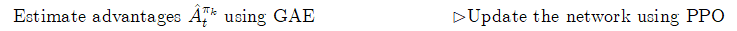参考博客列表：

Algorithmic和Algorithm环境_辉的博客-CSDN博客

LaTeX基本技巧_gsgbgxp的博客-CSDN博客_latex state

展开全文• latex 伪代码示例

## 例一：最常见的

\def\SetClass{article}
\documentclass{\SetClass}
\usepackage[ruled,linesnumbered]{algorithm2e}
\begin{document}
\begin{algorithm}
\caption{Simulation-optimization heuristic}\label{algorithm}
\KwData{current period $t$, initial inventory $I_{t-1}$, initial capital $B_{t-1}$, demand samples}
\KwResult{Optimal order quantity $Q^{\ast}_{t}$}
$r\leftarrow t$\;
$\Delta B^{\ast}\leftarrow -\infty$\;
\While{$\Delta B\leq \Delta B^{\ast}$ and $r\leq T$}{$Q\leftarrow\arg\max_{Q\geq 0}\Delta B^{Q}_{t,r}(I_{t-1},B_{t-1})$\;
$\Delta B\leftarrow \Delta B^{Q}_{t,r}(I_{t-1},B_{t-1})/(r-t+1)$\;
\If{$\Delta B\geq \Delta B^{\ast}$}{$Q^{\ast}\leftarrow Q$\;
$\Delta B^{\ast}\leftarrow \Delta B$\;}
$r\leftarrow r+1$\;}
\end{algorithm}
\end{document}

如图所示：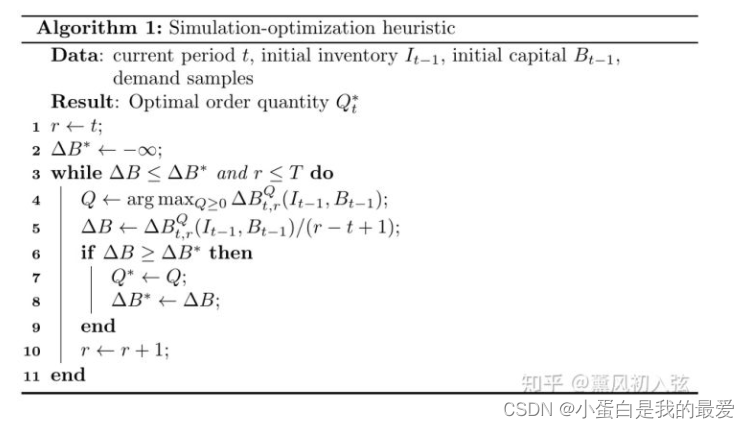例二：

\def\SetClass{article}
\documentclass{\SetClass}
\begin{document}
\IncMargin{1em}
\begin{algorithm}
\SetKwData{Left}{left}\SetKwData{This}{this}\SetKwData{Up}{up}
\SetKwFunction{Union}{Union}\SetKwFunction{FindCompress}{FindCompress}
\SetKwInOut{Input}{input}\SetKwInOut{Output}{output}

\Input{A bitmap $Im$ of size $w\times l$}
\Output{A partition of the bitmap}
\BlankLine
\emph{special treatment of the first line}\;
\For{$i\leftarrow 2$ \KwTo $l$}{
\emph{special treatment of the first element of line $i$}\;
\For{$j\leftarrow 2$ \KwTo $w$}{\label{forins}
\Left$\leftarrow$ \FindCompress{$Im[i,j-1]$}\;
\Up$\leftarrow$ \FindCompress{$Im[i-1,]$}\;
\This$\leftarrow$ \FindCompress{$Im[i,j]$}\;
\If(\tcp*[h]{O(\Left,\This)==1}){\Left compatible with \This}{\label{lt}
\lIf{\Left $<$ \This}{\Union{\Left,\This}}
\lElse{\Union{\This,\Left}}
}
\If(\tcp*[f]{O(\Up,\This)==1}){\Up compatible with \This}{\label{ut}
\lIf{\Up $<$ \This}{\Union{\Up,\This}}
\tcp{\This is put under \Up to keep tree as flat as possible}\label{cmt}
}
}
\lForEach{element $e$ of the line $i$}{\FindCompress{p}}
}
\caption{disjoint decomposition}\label{algo_disjdecomp}
\end{algorithm}\DecMargin{1em}
\end{document}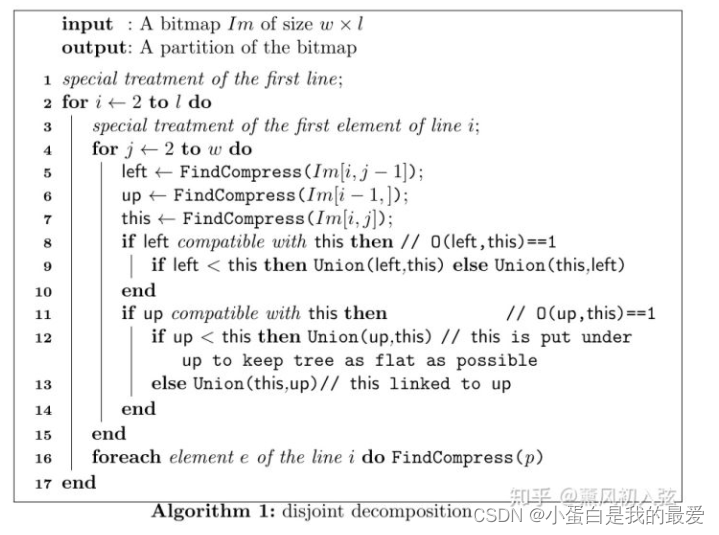展开全文大数据
• 这里我用到了Texstudio，大家戳下面的链接按照步骤进行下载：CSDN：最简单傻瓜式的 Latex+Texstudio 安装教学https://blog.csdn.net/qq_33826564/article/details/81490478Latex入门级：下载官方模板，在Texstudio/...
• then 肯定语句 else 否定语句 end 如果你不想让你的伪代码叫做 ‘Algorithm 编号’， 可以使用 \renewcommand{\algorithmcfname}{算法名} 命令来修改。 除了\If, \Else, \ElseIf之外，还有\uIf, \lIf, \uElse, \...
• latex在同时嵌套algorithm和algorithmc包之后，会出现伪代码标题序号不能自定义，只能algorithm1、algorithm2这样 二、解决方案 为了解决这个问题，需要加入下述语句 \renewcommand{\thealgorithm}{2-2} 具体位置...
• ## 5. LaTeX 算法伪代码基本格式排版

千次阅读 多人点赞 2021-11-20 00:34:12
LaTeX 算法伪代码基本格式排版 1、算法排版初体验 LaTeX 的所有宏包文件都要写在 \documentclass 命令之后和\begin{document} 之前。在本文中，我们主要会用到如下的两个宏包： \usepackage{algorithmic} \...
• ## Latex中加入算法伪代码模板

万次阅读 多人点赞 2018-05-28 16:55:15
写论文经常需要加入伪代码伪代码Latex中是一个模块，需要先引用几个包，然后在使用相应的指令来写： 1、在Latex头部加入以下包的引用： \usepackage{algorithm} \usepackage{algpseudocode} \usepackage{...
• Latex中加入算法伪代码模板 1.加入包，一定放在 \begin{document}之前 \usepackage{algorithm} \usepackage{algpseudocode} \usepackage{amsmath} \renewcommand{\algorithmicrequire}{\textbf{Input:}} \...
• 下面是伪代码的通用模板，我们先体验一下其效果，然后在后面详细讲解模板中的每个细节。 \begin{algorithm}[!h] \caption{Algorithm of SUM} \label{alg:AOS} \renewcommand{\algorithmi...
• \usepackage[ruled,linesnumbered]{algorithm2e} \begin{algorithm} \caption{pseudocode}\label{pseudocode} \SetKwInOut{Input}{input}\SetKwInOut{Output}{output} \Input{input data} \Output{output, if return...
• 这是一个LaTeX模板打开之后可以直接用的。然后只需要在这个模板基础之上编写自己的代码就OK了。
• right=2cm,top=1cm,bottom=1cm} % \linespread{1.5}\selectfont% 设置1.5倍行距 % =================== 中文伪代码特色 ========================== % \renewcommand{\algorithmcfname}{算法4 -} %细节与重点 \...linux windows 运维
• 1. 算法伪代码 \documentclass[AMA,STIX1COL]{WileyNJD-v2} \usepackage{moreverb} \begin{algorithm}[p] \small \caption{$ASMVP_{distr-LSH}$} \label{alg:Framwork} \begin{algorithmic} %这个1 表示每一...经验分享
• %if elseif else \If {"condition"} "text" \ElsIf {"condition"} "text" \Else "text" \EndIf LaTeX伪代码编辑模板 – OmegaXYZ ...
• LATEX——algorithm2e 伪代码书写模板 在为代码的各种模板中，algorithm2e的效果看起来比较舒服，更加简洁。 首先给出例子： \begin{algorithm}[t] \caption{example} \label{example} \begin{small} \BlankLine \...
• 在线LaTeX伪代码编辑网址https://www.sharelatex.com/project/59f985ff88ec741052f6394b
• 注释1：本文总体上关于文字排版软件latex的入门使用，顺便记录一下自己不算糟糕的科研生活，再顺便自己下此用到的时候方便查看.注释2：文采太差，小时候写日记我爸就说我记流水账，现在仍然学不会花哨的叙事方式，就...
• 0 写在前面 真是关关难过关关过，太讨厌转模板了，一下两天就过去了，要赶在周二玄学基础上，所以今天浅浅...我在这里主要是伪代码出现了错误，于是我先是添加了package，伪代码三件套 \usepackage{algorithm} \usepa深度学习
• latex取消伪代码的行号 只需要将\begin{algorithmic}后面的改成即可算法
• 当然，因为老师下发的模板是word的，而我又不可能为了一份大作业制作一份latex高仿的模板，所以最后还得去把tikz生成的pdf转换成svg之类的矢量图插入到word里，具体操作有空再填坑，暂且不表。以下是效果图...
• ## IEEE的Latex模板中伪代码和画图

千次阅读 多人点赞 2020-05-12 09:33:33
用IEEE的模板写论文时在算法流程图这卡住了，和一般的algorithm不同，需要\begin{figure} ， 这里贴几个可用的模板 \usepackage{algorithmic} \makeatletter \newcommand{\removelatexerror}{\let\@latex@error\...
• \KwOut{how to write algorithm with \LaTeX2e } initialization\; \While{not at end of this document}{ read current\; \eIf{understand}{ go to next section\; current section becomes this one\; }{ go...
• Word中使用Aurora插件插入latex伪代码下载以及配置word中的Aurora下载Aurora插件下载latex工具配置latex路径使用 下载以及配置word中的Aurora 下载Aurora插件 首先需要下载Aurora插件，下载地址： 链接：...
• \usepackage[ruled,linesnumbered]{algorithm2e} \usepackage{algpseudocode} \makeatletter \newcommand{\removelatexerror}{\let\@latex@error\@gobble} \makeatother %中略 \begin{figure}[!t] \...
• \renewcommand{\algorithmicrequire}{\textbf{Input:}} % Use Input in the format of Algorithm \renewcommand{\algorithmicensure}{\textbf{Output:}} % Use Output in the format of Algorithm ...
• 伪代码代码块 \begin{algorithm}[!h] \caption{PARTITION$(A,p,r)$}%算法标题 \begin{algorithmic}%一行一个标行号 \STATE $i=p$ \FOR{$j=p$ to $r$} \IF{$A[j]<=0$} \STATE $swap(A[i],A[j])$ \......